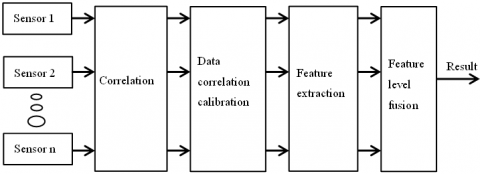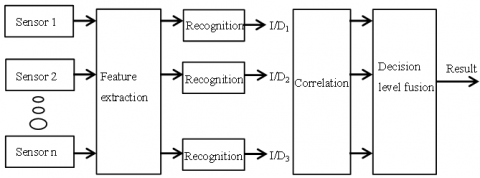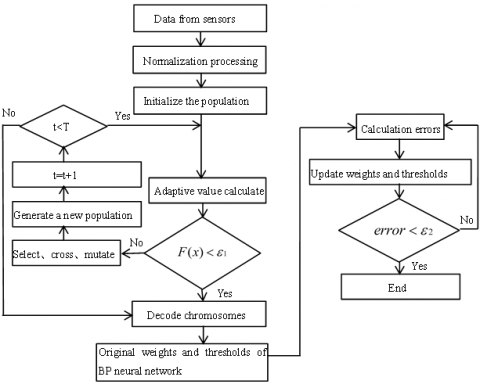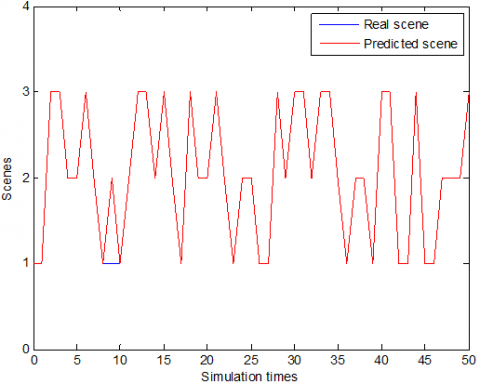# BP-GA Data Fusion Algorithm Studies Oriented to Smart Home

BP-GA Data Fusion Algorithm Studies Oriented to Smart Home

Tichun WangYuzhu Xie

College of Mechanical and Electrical Engineering, Nanjing University of Aeronautics and Astronautics, Nanjing 210016, China

Corresponding Author Email:
wangtichun2010@nuaa.edu.cn
Page:
135-140
|
DOI:
https://doi.org/10.18280/mmep.030304
| |
Published:
30 September 2016
| Citation

OPEN ACCESS

Abstract:

Data fusion is one of the key technologies in wireless sensor network technology. This paper studied a date fusion algorithm oriented to smart home through analyzing the function and operating feature of smart home appliances. Proposed BP-GA algorithm -- back propagation neural network optimized by genetic algorithm, and discussed its implementation process. This algorithm unites the advantages of BP neural network algorithm’s local search capability with genetic algorithm’s global search capability and fast convergence speed. Suboptimal solutions obtained by using the improved genetic algorithm are used as initial values of BP neural network algorithm. This can ensure rapidly global convergence of this BP neural network. Train this BP neural network to get the optimal solutions. Finally, smart lighting system is used to verify the algorithm.

Keywords:

Data fusion, Smart home, BP neural network, Genetic algorithm, Wireless sensor network

1. Introduction

With the development of science and economy, traditional discrete home appliances can’t meet people’s life demands anymore. So, many enterprises began to develop interrelated smart home appliances. Smart home appliances are controlled uniformly by the terminal, such as mobile phone, computer and remote control. They are interrelated and can communicate with each other. The wireless sensors  distributed in the building is the foundation of the smart home appliances. They can collect information about the surrounding environment automatically, perform date fusion , and make intelligent decision [2-3]. Data fusion is the core of smart home appliances. Too much time consuming is one of the important factors affecting intelligent level of smart home. So far, there have been a lot of researchers studying data fusion algorithm. However, these studies lack of general applicability  analysis to smart home appliances. The data fusion algorithm may be fast, high accuracy for one kind of smart appliances, while it is quite opposite for another kind of smart appliances. Thus the overall data fusion effect may not be positive. This paper analyzed functions and operating feature of smart home appliances, and proposed a date fusion algorithm oriented to smart home appliances specially. Improve encoded mode, use improved genetic algorithm to optimize BP neural network, and enhance data fusion general applicability. Finally, smart lighting system is used to verify this BP-GA algorithm.

2. The Function Model of Data Fusion

Data fusion is the process of sensor information data association, correlation, estimation and combination, to obtain target parameters, features, events, behavior description and identity estimation. It can be divided into three levels according to information abstraction degree: data level fusion, feature level fusion and decision level fusion [5-6].

2.1 Data level fusion

Data level fusion is the simplest fusion. External environment information data collected by multi sensors is fused to a very small degree. Get the result of data fusion from feature extraction and attribute judgment to the information. The advantages of data level fusion are best accuracy of fusion result, little information loss, and more intuitive and comprehensive cognition for people. Disadvantages are large amount of data processing, poor anti-interference ability, and there is no uniform method of data level fusion to fuse data from different type of sensors. As shown in figure 2-1.

## 1.pngFigure 2-1. Data level fusion

2.2 Feature level fusion

Feature level fusion is an intermediate level fusion . Correlate external environment information data collected by multi sensors. Then extract features of these information data. Finally, feature layer fusion. Feature level fusion can preserve important characteristics of target, diminish information volume and improve real-time performance of information processing. However, information capacity decrease also leads to some information loss.

## 2.pngFigure 2-2. Feature level fusion

2.3 Decision level fusion

Decision level fusion is the highest level fusion . Extract features of external environment information data collected by multi sensors. Then estimate identities of these features respectively. The information collected by each sensor is estimated respectively. Finally, different decision results are fused according to relevant decision criteria. According to specific decision requirements, decision level fusion selects appropriate fusion algorithm to fuse the results obtained from feature level fusion. Decision level fusion has a good real-time performance, strong anti - interference ability and flexibility. It can be applied to heterogeneous sensors, and it can work normally when one or more sensors fail. Disadvantage is the large amount of information loss. As shown in figure 2-3.

## 3.pngFigure 2-3. Decision level fusion

3. The Structure of BP-GA Algorithm Oriented to Smart Home

Smart home is based on home, using a variety of technologies to integrate home life related facilities, building efficient, comfortable, safe and convenient home environment. Smart home system provides many kinds of functions and methods such as appliances control, lighting control, telephone remote control, indoor and outdoor remote control, anti-theft alarm, environmental monitoring, HVAC control, infrared transmitting and programmable timing control. Compared with ordinary home, smart home not only has traditional residential functions, but also has building, network communications, information appliances and equipment automation, providing a full range of information interaction capabilities. Data fusion is the core of smart home system. Sensors in smart home system are placed in an overlapping way. Information collection is more comprehensive, while it leads to data redundancy in the network. The computing power and communication ability of sensor nodes are limited, so data fusion technology is needed to eliminate data redundancy, save communication energy consumption, and enhance timeliness and accuracy of the system. In this paper, genetic algorithm is used to optimize BP neural network algorithm for data fusion, which can not only deal with the nonlinear smart home control system, but also improve the scalability and real-time performance of the system.

3.1 Genetic algorithm optimizes thresholds and weights of BP neural network

Genetic algorithm is an adaptive global optimization search algorithm . It searches for the optimal solution through simulating biological selection, crossover, replication and gene mutation, not relay on specific issues. First abstract a population with potential solution set from the problem domain. Encode the population into a fixed number of individual set with characteristic chromosomes by gene coding . Then select, cross, replicate and mutate these chromosomes to evolve a new solution population until the optimal solution is founded.

3.1.1 Optimize coding mode

Set chromosome number to$N$. Arrange weights and thresholds of BP neural network $Wij$、$Vij$、$\theta j$、$\gamma t$into a long string according to a certain order. Encoding in this way is much better than basic binary code, and this is an improvement of the simple genetic algorithm (SGA). This is because the weight learning is a complex parameter optimization problem. General binary code makes element string overlong . The result needs to be decoded into real number after the training. This greatly affects the efficiency and accuracy of network training.

3.1.2 Optimize genetic operators

Due to the encoding change of weights and thresholds, the corresponding genetic operators (crossover and mutation) should do adaptive adjustment too. Choosing crossover probability and mutation probability reasonably and accurately is an important factor to affect the efficiency of the simple genetic algorithm, but there is still no theoretical basis for reasonable probability choice. It only provides a recommended selecting range, among them, $pc\in (0.40,0.99)$,$pm\in (0.0001,0.1)$. This paper proposes the methods of adaptive crossover probability and adaptive mutation probability to operate the coding string. The improved formulas are as follows:

$pc=\left\{ \begin{matrix} C{{(f\max -fc)}^{2}}/{{(f\max -\overline{f})}^{2}}, & fc\ge \overline{f} \\ C, & fc<\overline{f} \\\end{matrix} \right.$      (1)

$pm=\left\{ \begin{matrix} M{{(f\max -fm)}^{2}}/{{(f\max -\overline{f})}^{2}}, & fm\ge \overline{f} \\ M, & fm<\overline{f} \\\end{matrix} \right.$     (2)

Among them, $C$ and $M$ are both constant less than 1. $fc$ is the larger adaptive value between the two gene strings to be crossed. $f\max$ is the largest adaptive value in the population. $\overline{f}$ is the average adaptive value in the population. $fm$ is the adaptive value of the string to mutate. $f\max -\overline{f}$ indicates convergence degree of the population. When its value is relatively small, it indicates that the population tends to convergent.

When genetic operators is finished, a new chromosome instead of the original one, and we can get a new population $N'$. If the optimal solution is obtained, decode the optimized parameter set (optimal chromosomes) and get the optimal weights and thresholds of the network to assign values to the initial weights and thresholds of BP neural network.

3.2 Data fusion algorithm of BP neural network

BP (Back-Propagation) neural network is currently one of the most popular and successful neural network models. As shown in figure 3-1, BP neural network includes input layer, hidden layer and output layer. There is no connection and no feedback between the neurons in the same layer. Neurons only connect with the one in adjacent layers, which form a feedforward neural network .

Data parameters in BP neural network transmit forward, While the computing errors follow back propagation. In the process of data parameters forward transmission, data passes through the input layer to the hidden layer, exchange information in the hidden layer, and finally output the information from the output layer. If output information does not match the expected information, it enters error back propagation stage. In the process of error back propagation, computing errors transmit to hidden layer and input layer in turn, modify weights and thresholds of the neural network. In the cyclic process of data parameters forward transmission and computing errors back propagation, computing errors are adjusted continuously according to the gradient descent, until the output information is in line with the expected value.

## 4.pngFigure 3-1. Structure diagram of BP neural network

3.2.1 Self-learning model of BP neural network

BP neural network self-learning process consists of two parts, data parameters forward transmission and errors back propagation. Its core is self-learning of network weights and thresholds. Suppose the input layer of BP neural network has $n$ nodes, the hidden layer has $p$ nodes, and the output layer has $m$ nodes. Set up a training sample $T$, which input signal is $x={{(x1,x2,....,xi,...,xn)}^{T}}$, expected output is .., weight and threshold of input layer to hidden layer are respectively $wij$, $\theta j$, and weight and threshold of hidden layer to output layer are respectively $wjk$, $\theta k$.

In the process of signal forward propagation, the outputs of node in each layer are:

$\left\{ \begin{matrix} x{{j}^{'}}=f(\sum\limits_{i=1}^{n}{wijxi-\theta j}), & j=1,2,...,p \\ yk=f(\sum\limits_{j=1}^{p}{wjkx{{j}^{'}}-\theta k}), & k=1,2,...,m \\\end{matrix} \right.$     (3)

The output function is sigmoid function:

$f(x)=\frac{1}{1+{{e}^{-x}}}$     (4)

Therefore, the output of hidden layer is ${{x}^{'}}={{(x{{1}^{'}},x{{2}^{'}},....,x{{j}^{'}},...,x{{p}^{'}})}^{T}}$, and the output of output layer is $y={{(y1,y2,....,yk,...,ym)}^{T}}$.

In the process of signal forward propagation, the network error function is:

$Ex'=\frac{1}{2}\sum\limits_{k=1}^{k=m}{{{(tk-yk)}^{2}}}$     (5)

According to the gradient descent method, suppose the variable of hidden layer to output layer is $\Delta Wjk$, that is:

$\Delta Wjk=-\eta \frac{\partial Ex'}{\partial Wjk},\eta >0$     (6)

For output layer:

$Wj{{k}^{'}}=Wjk-\eta \frac{\partial Ex}{\partial Wjk}$     (7)

For hidden layer:

$Wi{{j}^{'}}=Wij-\eta \frac{\partial Ex}{\partial Wij}$     (8)

Recalculate signal and error of hidden layer and output layer according to the corrected weights and thresholds. Repeat this process. The training will not finish until the outputs are desirable.

3.3 Algorithm process

Firstly, use the improved genetic algorithm to obtain suboptimal solutions. Then, use these solutions as the initial values of BP neural network algorithm for training. It can not only ensure global convergence of BP neural network, but also improve the training speed and convergence accuracy of smart home system.

The key steps of BP-GA algorithm are as follows:

(1) Initialize population. Set the chromosome number as $N$, set evolution generations as$T$, and set error precision as $\varepsilon 1$. Combine the weights and thresholds of BP neural network $wij$, $\theta j$, $wjk$, $\theta k$ into a long string. According to the order, the four positions on the string correspond to different weights and thresholds.

Real-number encoding is chosen as the coding mode, because traditional binary code makes element string overlong, and the evolutionary results need to be decoded into real number after the training, which greatly affects the efficiency and precision of network training. Encoding length is:

$S=np+pm+p+m$     (9)

Among them, $n$is the node number of input layer, $p$ is the node number of hidden layer, and $m$ is the node number of output layer.

(2) Calculate the adaptive value of each chromosome. If the adaptive value is less than $\varepsilon 1$, decode the chromosome to get sub-optimal weights and threshold; otherwise, go to step 3. The adaptive function is the reciprocal of the square sum of the neural network errors.

$f(x)=\frac{1}{\sum\limits_{i=1}^{i=T}{{{(t(x)i-y(x)i)}^{2}}}}$      (10)

Among them, $t(x)i$ is the expectancy output, and $y(x)i$ is the actual output. Since the smaller the error between expectancy output and actual output, the better, the possibility of a chromosome to be chosen is larger when its adaptive value is larger.

(3) Selection operator. Roulette selection is chosen as section operator. The larger the adaptive value of the chromosome, the greater the probability of being chosen to the next generation.

$p(xi)=\frac{f(xi)}{\sum\limits_{i=1}^{i=T}{(f(xi))}}$     (11)

(4) crossover operator and mutation operator. Confirm crossover probability $pc$and mutation probability $pm$. Chromosomes are matched by random number 0-1 to carry on chromosome crossover and evolution. The evolutionary generation increases one after finishing a round of genetic operation.

(5) If the evolutionary generation $t<T$, turn to step 2, otherwise decode chromosomes to get suboptimal weights and thresholds of BP neural network.

(6) obtain original weights and thresholds of BP neural network by genetic algorithm. Set the accuracy of BP neural network as $\varepsilon 2$.

When the network error is less than the preset precision $\varepsilon 2$ after training, the predicted output value is the expectancy output value. The process of GA-BP algorithm is shown as figure 3-2.

## 5.pngFigure 3-2. The process of GA-BP algorithm

4. Simulation and Verification

In order to check the effectiveness of the GA-BP algorithm, this paper uses R2014a Matlab to write the algorithm program to simulate and verify the algorithm. Place 3 sensor nodes at the right positions of a 5m * 5m room, and then let 1 to 7 persons in randomly to test it.

There are three inputs in GA-BP neural network  (data acquired from infrared sensors), and input variables are real number. There are three variables in the output node, and output variables are binary variables (0 and 1). Among the three output variables, there is one and only one variable being 1, others are 0. The node which output variable is 1 corresponds to corresponding smart lighting scene. (e.g. 001 corresponds to the scene of “home back”- porch lights are turned on with 100% light brightness. Then living room lights, stair lights, bedroom lights, and bathroom lights are turned on in turn. The lights in a room with no one to enter in ten seconds will go out automatically. 010 corresponds to the scene of “welcome”- porch lights are turned on with 100% light brightness. Then living room lights and stair lights are on in turn. The lights in a room with no one to enter in ten seconds will go out automatically. 100 corresponds to the scene of “party”- porch lights and living room lights are turned on at the same time with 70% light brightness, springing to flash, color gradient.

Simulation program selected 100 group of data to normalize, and selected 50 group of data from them randomly as training samples. Sample training is the process of weights and thresholds adjustment. The data set of weights and thresholds is just the parameters of GA-BP algorithm of smart light system. The other 50 group of data were used for investigating generalization ability of GA-BP algorithm. Population size of improved genetic algorithm is $N=9$, training time of BP neural network is $L=50$, evolution generation is $K=80$, selection probability is $ps=0.5$, crossover probability is $pc=0.9$, and mutation probability is $pm=0.01$. When the error of GA-BP algorithm is less than 0.002, finish the training. The output weights and the thresholds at this time reach global optimal values.

## 6.pngFigure 4-1. Scenes output of traditional BP neural network algorithm

## 7.pngFigure 4-2. Scenes output of BP-GA neural network algorithm

From figure 4-1 and table 2 we can see that the traditional BP neural network algorithm has an error rate of 10 %. Its training time is larger and its convergence rate is small compared to BP-GA algorithm. From figure 4-2 and table 2 we can see that the error rate of BP-GA algorithm is about 2 %. This is a huge improvment. Also, its  training time is decreased and its convergence rate is incresed. The experimental result shows that, GA-BP algorithm improves the correctness and efficiency of data fusion in smart home.

Table 1. Part of the test samples

 No. Sensor 1 Sensor 2 Sensor 3 Actual number Scene category 1 1 1 0 1 1 2 0 1 1 1 1 3 2 2 1 2 2 4 7 6 5 7 3 5 3 2 2 3 2 6 5 5 6 6 3 7 3 4 3 4 2 8 4 5 4 5 3 9 2 3 3 3 2 10 2 1 1 2 1

Table 2. Comparison of algorithm performances

 Algorithm Average training times Average convergence  rate (s) Accuracy Traditional BP 42 8.13 90 % Improved GA-BP 34 4.31 98 %.
5. Conclusions

This paper analyzes the problems existing in data fusion algorithm for smart home, and offers the structure of the  effective algorithm for smart home. Aiming at the problem of time consuming and general applicability of data fusion in smart home, this paper proposes using genetic algorithm to optimize the BP neural network and using real number encoding to improve the simple genetic algorithm. Finally, intelligent lighting system is used as an example to verify the effectiveness of BP-GA algorithm. The experimental result shows that, GA-BP algorithm can reduce time consuming and improve the fusion precision in smart home.

Acknowledgment

This research was supported by the National Natural Science Foundation Youth Fund of China (No. 51005114); The Fundamental Research Funds for the Central Universities, China (No. NS2014050); The Research Fund for the Doctoral Program of Higher Education，China(No. 20112302130003); Jiangsu Planned Projects for Postdoctoral Research Funds (No. 1301162C).

References

 Sun Limin, Li Jianzhong, Chen Yu, et al., Wireless Sensor Networks, Beijing: Publishing House of Tsinghua University, 2005, pp. 1-2.

 Zhou Fang and Han Liyan, “Review of multi sensor information fusion technology,” Telemetry and Remote Control, vol. 27, no. 3, pp. 1-7, 2006.

 Lu Manli and Sun Lingfang, “Multi sensor information fusion technology,” Automation Technology and Application, vol. 27, no. 2, pp. 221-224, 2008.

 Liu Longqing, “Research of intelligent lighting control system based on wireless sensor / actuator network,” M.S. thesis, South China University of Technology, Nanjing, China, 2014

 Kang Jian, Zuo Xianzhang, Tang Liwei, Zhang Xihong and Li Hao, “Data fusion technology for wireless sensor networks,” Computer Science, no. 04, pp. 31-35 and 58, 2010.

 Huang Manguo and Fan Shangchun, Zheng Dezhi, Xing Weiwei, “Research progress of multi-sensor data fusion technology,” Sensor and Micro System, no. 03, pp. 5-8 and 12, 2010.

 Wu Yan, “Multi sensor data fusion algorithm research,” M.S. thesis,  Xi’an Electronic and Science University, Xi’an, China, 2003.

 Chen Zhengyu, Yang Geng, Chen Lei and Xu Jian, “Research on data fusion in wireless sensor networks,” Computer Application Research, no. 05, pp. 1601-1604 and 1613, 2011.

 Ma Yongjie and Yun Wenxia, “Research progress of genetic algorithm,” Computer application research,04, pp. 1201-1206 and 1210, 2012.

 Bian Xia and Mi Liang, “Research progress of genetic algorithm theory and its application,” Computer Application Research,07, pp. 2425-2429 and 2434, 2010.

 Cao Daoyou, “Application Research Based on improved genetic algorithm,” M.S. thesis, Anhui Univ., Hefei, China, 2010.

 Li Youkun, “BP neural network analysis and improvement of its application of,” M.S. thesis, Anhui University of Science And Technology, Chuzhou, China, 2012.

 Li Zong, “Research on lighting control system in smart home,” M.S. thesis, Shanghai Jiao Tong Univ., Shanghai, China, 2008.